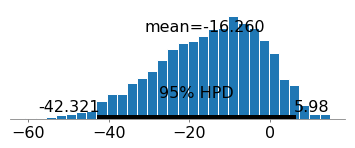### Bayesian Logistic Regression in Python using PYMC3In my last post I talked about bayesian linear regression. A fairly straightforward extension of bayesian linear regression is bayesian logistic regression. Actually, it is incredibly simple to do bayesian logistic regression. If you were following the last post that […]